Example Questions

← Previous 1

Example Question #6 : Squares

ABCD and EFGH are squares such that the perimeter of ABCD is 3 times that of EFGH. If the area of EFGH is 25, what is the area of ABCD?

75

225

5

25

15

225

Explanation:

Assign variables such that

One side of ABCD = a

and One side of EFGH = e

Note that all sides are the same in a square. Since the perimeter is the sum of all sides, according to the question:

4a = 3 x 4e = 12e or a = 3e

From that area of EFGH is 25,

e x e = 25 so e = 5

Substitute a = 3e so a = 15

We aren’t done. Since we were asked for the area of ABCD, this is a x a = 225.

Example Question #7 : Squares

A square has an area of 36. If all sides are doubled in value, what is the new area?

48

108

144

72

132

144

Explanation:

Let S be the original side length. S*S would represent the original area. Doubling the side length would give you 2S*2S, simplifying to 4*(S*S), giving a new area of 4x the original, or 144.

Example Question #9 : Squares

Freddie is building a square pen for his pig. He plans to buy x feet of fencing to build the pen. This will result in a pen with an area of p square feet. Unfortunately, he only has enough money to buy one third of the planned amount of fencing. Which expression represents the area of the pen he can build with this limited amount of fencing?

3p

p/6

p/3

p/9

9p

p/9

Explanation:

If Freddie uses x feet of fencing makes a square, each side must be x/4 feet long. The area of this square is (x/4)2 = x2/16 = p square feet.

If Freddie uses one third of x feet of fencing makes a square, each side must be x/12 feet long. The area of this square is (x/12)2x2/144 = 1/9(x2/16) = 1/9(p) = p/9 square feet.

Alternate method:

The scale factor between the small perimeter and the larger perimeter = 1 : 3. Since we're comparing area, a two-dimensional measurement, we can square the scale factor and see that the ratio of the areas is 1: 32 = 1 : 9.

Example Question #1 : How To Find The Area Of A Square

If the diagonal of a square measures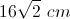, what is the area of the square?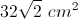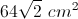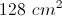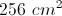Explanation:

This is an isosceles right triangle, so the diagonal must equal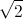times the length of a side. Thus, one side of the square measures, and the area is equal to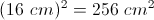Example Question #2 : How To Find The Area Of A Square

A square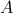has side lengths of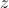. A second square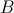has side lengths of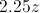. How many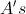can you fit in a single?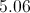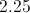Explanation:

The area ofis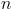, the area ofis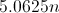. Therefore, you can fit 5.06in.

Example Question #3 : How To Find The Area Of A Square

The perimeter of a square is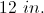If the square is enlarged by a factor of three, what is the new area?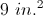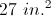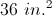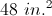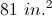Explanation:

The perimeter of a square is given by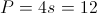so the side length of the original square is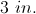The side of the new square is enlarged by a factor of 3 to give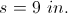So the area of the new square is given by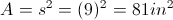.

Example Question #4 : How To Find The Area Of A Square

A half circle has an area of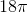. What is the area of a square with sides that measure the same length as the diameter of the half circle?

36

108

81

144

72

144

Explanation:

If the area of the half circle is, then the area of a full circle is twice that, or.

Use the formula for the area of a circle to solve for the radius:

36π = πr2

r = 6

If the radius is 6, then the diameter is 12. We know that the sides of the square are the same length as the diameter, so each side has length 12.

Therefore the area of the square is 12 x 12 = 144.

Example Question #5 : How To Find The Area Of A Square

In square WXYZ, point Q is the midpoint of side WZ.  If the area of quadrilateral WXYQ is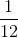, what is the length of one side of square WXYZ?Explanation:

Letequal the length of one side of the square.  Drawing a diagram yields WXYQ is a trapezoid with two right angles, parallel bases ofand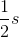, and a height of.

Area of WXYQ =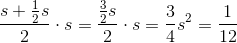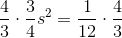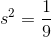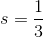Example Question #6 : How To Find The Area Of A Square

Four perfect circles with equal dimensions fit perfectly in a square with a length and width of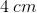. What is the area of the space inside the square that does not include the circles? (Use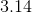for the value of.)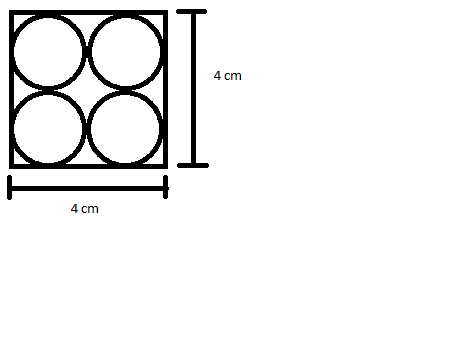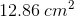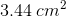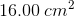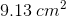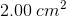Explanation:

Solving for the area not occupied by circles involves subtracting the area of all four circles from the total area of the square, so let's start by calculating the area of the square. We are told that it has a length and width of, so we just need to multiply these together: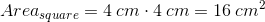Now, we need to calculate the area of one of the circles. Since the four circles fit perfectly into the square, each one has a diameter of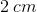and a radius of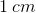, because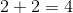and the square's sides are eachlong.

Knowing this, we can calculate the area of one of the circles using the equation for the area of a circle,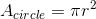. Substituting infor the value of the radius of one of the circles, we get: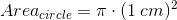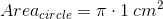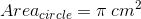Now, we can multiply our result byto calculate the area encompassed by the four circles together and subtract that value from the area of the square to find the answer: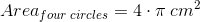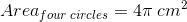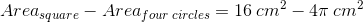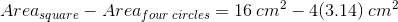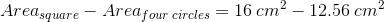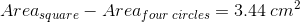Example Question #7 : How To Find The Area Of A Square

Find the area of a square with side length 4.Explanation:

To solve, simply use the formula for the area of a square.

Substitute the side length of four into the following equation.

Thus,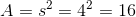← Previous 1